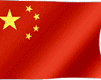中文

Design the Spur gear

Step 1
Obtain the required application data module, P
Teeth, N
Pressure angle, PA
Tooth width, f (inches)
Input speed, n (RPM)
Input torque, T I or type horsepower, HP I step 2
Obtain data and correction factors derived pitch circle diameter, D P = N / P
Tooth factor, y
Yield stress, S b working life factor, C S rate factor, C V material strength factor, C m temperature correction coefficient, C T

◆ Ambient temperature <100oF, C T = 1
◆ 100oF <ambient temperature <200oF
C T = 1/1 (1 + α (T-100oF))
Here, α = 0.022 corresponds MC Nylon
α = 0.004 corresponds nylon 101
α = 0.010 corresponds POM

Step 3
Using equation (1) and (2) calculation of the maximum torque or horsepower

T MAX = D P S b fyC s C v C m C T / 2P (1)

HP MAX = D P S b fyn C S C V Cm C T / 126000 P (2)
Step 4
The maximum torque (T MAX) and the maximum horsepower (H MAX) with known plastic gears input torque (Ti) and horsepower (Hi) to compare.
Ti must be less than or equal to T MAX Hi must be less than or equal to H MAX such as plastic gears Hi and T I than T MAX and H MAX, choose another material or another of the pitch circle diameter and tooth thickness, in accordance with the new material amendment coefficient recalculated.